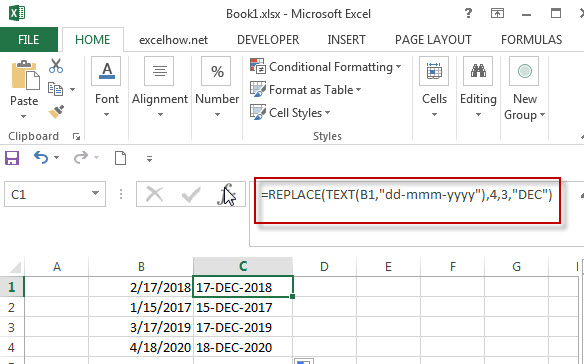# Combining the Replace function with Text function in Excel

In excel, we can use Replace function in combination with Text function to replace date characters that are part of the text string. And this post will guide you how to use TEXT function as the first arguments to replace text string in REPLACE function in excel 2013.

We have talked in the previous post that the TEXT function will return a text string, so the returned result of Text function can be as the first argument in the REPLACE function.

The Excel REPLACE function replaces all or part of a text string with another text string

The syntax of the REPLACE function is as below:

`= REPLACE(old_text, start_num, num_chars, new_text)`

## Combining the Replace function with Text function

Assuming that there is a date list in Column B, and you want to replace all month name to “DEC”.  As the date is recognized as numeric value in excel, you must convert the date as text string using TEXT function.  So we can write down the following REPLACE function:

`=REPLACE(TEXT(B1,”dd-mmm-yyyy”),4,3,”DEC”)`

We can enter the above REPLACE formula into Cell B1, then drag the Fill Handle to the entire Column B. it will apply for the other cells for this formula. Then you will get the expected results. Let’s see the following screenshot:### Related Functions

• Excel Replace function
The Excel REPLACE function replaces all or part of a text string with another text string.The syntax of the REPLACE function is as below:=REPLACE (old_text, start_num, num_chars, new_text)…
• Excel Text function
The Excel TEXT function converts a numeric value into text string with a specified format. The TEXT function is a build-in function in Microsoft Excel and it is categorized as a Text Function. The syntax of the TEXT function is as below: = TEXT (value, Format code)…